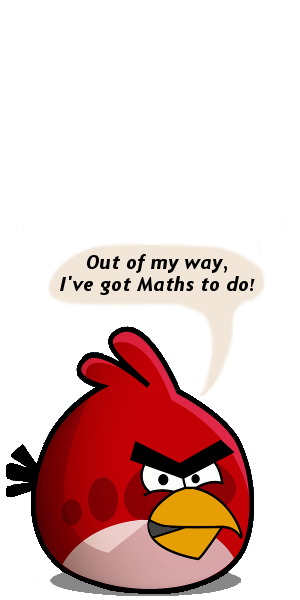# Year 9 On-line

The IXL site is good in that it makes questions progressively harder when students answer correctly, but unfortunately it is written for the US curriculum, so sometimes isn't appropriate for NZ students.

## Number I

MathsBuddy
Number – Decimals, Integers, The Number System I and II (only up to lesson 8)

nz.ixl.com
Number Theory: A.1, A.3–A.7
Integers: B.1, B.3
Operations with Integers: C.1–C.7
Rational Numbers: D.2, D.4, D.6, D.7, D.8
Operations with Rational Numbers: E.2–E.6
Exponents and Roots: F.1–F.5, F.15

www.thatquiz.org
Concepts – Place value: especially rounding of decimals
Geometry – Number line: include negatives and decimals
Integers – Inequality: include all the operations and negatives
Integers – Factors: include all options on the left

## Number II

MathsBuddy
Number – Fractions, Percentages, Calculator and Percentages
Year 10 Number – Ratio, Rates

nz.ixl.com
Percents: J.1–J.11
Consumer Maths: K.4–K.5 (K.6–K.8 are extension)
Ratios and Proportion: H.1–H.9 (H.10–H.13 are extension)

www.thatquiz.org
Fractions – Simplify: tick all the possible conversion options
Fractions – Inequality: tick all the possible conversion options
Fractions – Arithmetic: include inverted and negatives

## Measurement

MathsBuddy
Measurement – Measurement Conversions
Measurement – Area, Volume, Measurement and the Circle

nz.ixl.com
Measurement: L.1–L.3 (L.4–L.6 are extension)
Geometry: P.13–P.15, P.17, P.24, P.25, P.28 (cones, pyramids etc are not required)

www.thatquiz.org
Concepts – Time: all options
Concepts – Measure
Geometry – Geometry: not spheres and cones ("solve x" is extension only)

## Algebra

MathsBuddy
Algebra – Algebra I and II: but not Completing Number Patterns
Algebra – Linear equations: up to 5. Two Step Equations (after that is extension)

nz.ixl.com
Variable expressions
Single-variable expressions: not U.6
Inequalities: extension only

www.thatquiz.org
Integers – Algebra: especially "Solve x", but "Solve y" and "Simplify" as well.

## Patterns and Graphs (Algebra II)

MathsBuddy
Algebra – Two-Dimensional Graphs: up to Lesson 3 "Graphs using Tables" only
Statistics – Representing Data: Travel Graphs only

nz.ixl.com
Number Sequences: R.2, R.5, R.7
Linear Functions: V.3–V.7 (V.8 and V.9 are extension)

www.thatquiz.org
Geometry – Points: only Cartesian, both plotting and identifying

## Shapes and Angles (Geometry I)

MathsBuddy
Geometry – Geometry in Two Dimensions: up to Lesson 9 "Special Triangles" only

nz.ixl.com
Geometry: P.4–P.8 (not hugely useful)

www.thatquiz.org
Geometry – Angles: tick all boxes

## Transformations (Geometry II)

MathsBuddy
Geometry – Transformations and Solids

nz.ixl.com
Transformations: up to Q.7 (note: dilation = enlargement, and is extension only)

## Statistics

While on-line resources are useful for testing statistics skills, the NZ curriculum increasingly tests meaning. Students need to not only know how to find a mean and median, but what they show about data.

MathsBuddy
Statistics – Representing Data: up to Lesson 4 "Pie Graphs"
Statistics – Statistics: up to Lesson 4 "Frequency Distribution Tables" (rest is extn)

nz.ixl.com
Statistics: all (but BB.3 and BB.6 are less useful)
Charts and Graphs: all except M.13 and M.15

www.thatquiz.org
Integers – Averages: all, but not very challenging
Concepts – Graphs: especially "Box Plot" and tick everything below the bar

## Probability

MathsBuddy
Statistics – Probability: all

nz.ixl.com
Probability: all, but most especially the first ones

www.thatquiz.org
Fractions – Probability: focused and not (get hard quite quickly)